# User:Tohline/VE/RiemannEllipsoids

(Difference between revisions)
 Revision as of 13:34, 4 August 2020 (view source)Tohline (Talk | contribs) (→The Six Off-Diagonal Elements)← Older edit Revision as of 13:42, 4 August 2020 (view source)Tohline (Talk | contribs) (→The Six Off-Diagonal Elements)Newer edit → Line 362: Line 362: +
[ [[User:Tohline/Appendix/References#EFE|EFE]], Chapter 7, §47, Eq. (3) ]
whereas for $~i=3$ and $~j=2$, whereas for $~i=3$ and $~j=2$, Line 375: Line 376:
$~ [itex]~ - 2 \mathfrak{T}_{ij} - \Omega_i\Omega_k I_{kj} + 2\epsilon_{ilm}\Omega_m \int_V \rho u_lx_j dx + 2 \mathfrak{T}_{32} - \Omega_3 \Omega_2 I_{22} + 2\Omega_2 \int_V \rho u_1x_2 dx + - 2\Omega_1 \cancelto{0}{\int_V \rho u_2 x_2 dx} \, , \, ,$ [/itex]
[ [[User:Tohline/Appendix/References#EFE|EFE]], Chapter 7, §47, Eq. (4) ]
+ - where, in both cases, we have acknowledged that, given the above specific prescription for the internal velocity field, $~\vec{u}$, + where in both cases we have acknowledged that, given the above specific prescription for the internal velocity field, $~\vec{u}$, Line 396: Line 399:
if     $~i = j \, .$       if     $~i = j \, .$
[ [[User:Tohline/Appendix/References#EFE|EFE]], Chapter 7, §47, Eq. (5) ]
+

# Steady-State 2nd-Order Tensor Virial Equations

By satisfying all six — not necessarily unique — components of the Second-Order Tensor Virial Equation, the entire set of Riemann Ellipsoids can be determined.

Here we are only interested in determining the equilibrium conditions of uniform-density ellipsoids that have semi-axes,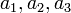$~a_1, a_2, a_3$.

## General Coefficient Expressions

As has been detailed in an accompanying chapter, the gravitational potential anywhere inside or on the surface,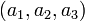$~(a_1,a_2,a_3)$, of an homogeneous ellipsoid may be given analytically in terms of the following three coefficient expressions: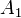$~A_1$$~=$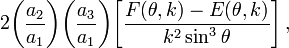$~2\biggl(\frac{a_2}{a_1}\biggr)\biggl(\frac{a_3}{a_1}\biggr) \biggl[ \frac{F(\theta,k) - E(\theta,k)}{k^2 \sin^3\theta} \biggr] \, ,$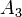$~A_3$$~=$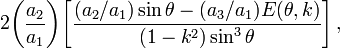$~2\biggl(\frac{a_2}{a_1}\biggr) \biggl[ \frac{(a_2/a_1) \sin\theta - (a_3/a_1)E(\theta,k)}{(1-k^2) \sin^3\theta} \biggr] \, ,$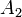$~A_2$$~=$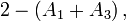$~2 - (A_1+A_3) \, ,$

where,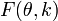$~F(\theta,k)$ and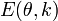$~E(\theta,k)$ are incomplete elliptic integrals of the first and second kind, respectively, with arguments,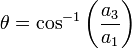$~\theta = \cos^{-1} \biggl(\frac{a_3}{a_1} \biggr)$ and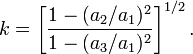$~k = \biggl[\frac{1 - (a_2/a_1)^2}{1 - (a_3/a_1)^2} \biggr]^{1/2} \, .$ [ EFE, Chapter 3, §17, Eq. (32) ]

EFE (p. 130) states that the … kinematical requirement, that the motion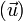$~(\vec{u})$, associated with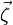$~\vec{\zeta}$, preserves the ellipsoidal boundary, leads to the following expressions for its components: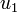$~u_1$$~=$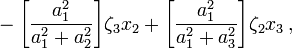$~- \biggl[ \frac{a_1^2}{a_1^2 + a_2^2}\biggr] \zeta_3 x_2 + \biggl[ \frac{a_1^2}{a_1^2+a_3^2}\biggr] \zeta_2 x_3 \, ,$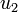$~u_2$$~=$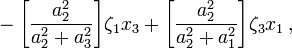$~- \biggl[ \frac{a_2^2}{a_2^2 + a_3^2}\biggr] \zeta_1 x_3 + \biggl[ \frac{a_2^2}{a_2^2+a_1^2}\biggr] \zeta_3 x_1 \, ,$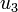$~u_3$$~=$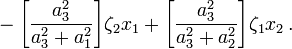$~- \biggl[ \frac{a_3^2}{a_3^2 + a_1^2}\biggr] \zeta_2 x_1 + \biggl[ \frac{a_3^2}{a_3^2+a_2^2}\biggr] \zeta_1 x_2 \, .$ [ EFE, Chapter 7, §47, Eq. (1) ]

## Equilibrium Expressions

[EFE §11(b), p. 22] Under conditions of a stationary state, [the tensor virial equation] gives,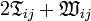$~2 \mathfrak{T}_{ij} + \mathfrak{W}_{ij}$$~=$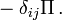$~- \delta_{ij}\Pi \, .$

[This] provides six integral relations which must obtain whenever the conditions are stationary.

When viewing the (generally ellipsoidal) configuration from a rotating frame of reference, the 2nd-order TVE takes on the more general form: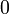$~0$$~=$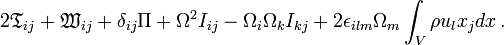$~ 2 \mathfrak{T}_{ij} + \mathfrak{W}_{ij} + \delta_{ij}\Pi + \Omega^2 I_{ij} - \Omega_i\Omega_k I_{kj} + 2\epsilon_{ilm}\Omega_m \int_V \rho u_lx_j dx \, .$ [ EFE, Chapter 2, §12, Eq. (64) ]

EFE (p. 57) also shows that … The potential energy tensor … for a homogeneous ellipsoid is given by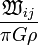$~\frac{\mathfrak{W}_{ij}}{\pi G\rho}$$~=$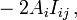$~-2A_i I_{ij} \, ,$ [ EFE, Chapter 3, §22, Eq. (128) ]

where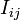$~I_{ij}$$~=$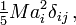$~\tfrac{1}{5} Ma_i^2 \delta_{ij} \, ,$ [ EFE, Chapter 3, §22, Eq. (129) ]

is the moment of inertia tensor.

### The Three Diagonal Elements

For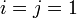$~i = j = 1$, we have,$~0$$~=$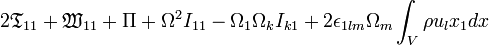$~ 2 \mathfrak{T}_{11} + \mathfrak{W}_{11} + \Pi + \Omega^2 I_{11} - \Omega_1\Omega_k I_{k1} + 2\epsilon_{1lm}\Omega_m \int_V \rho u_lx_1 dx$$~=$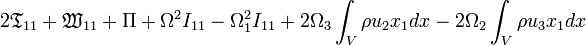$~ 2 \mathfrak{T}_{11} + \mathfrak{W}_{11} + \Pi + \Omega^2 I_{11} - \Omega_1^2I_{11} + 2 \Omega_3 \int_V \rho u_2x_1 dx - 2\Omega_2 \int_V \rho u_3x_1 dx$$~=$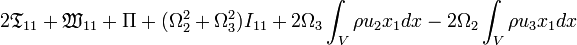$~ 2 \mathfrak{T}_{11} + \mathfrak{W}_{11} + \Pi +( \Omega_2^2 + \Omega_3^2) I_{11} + 2 \Omega_3 \int_V \rho u_2x_1 dx - 2\Omega_2 \int_V \rho u_3x_1 dx$

Similarly, for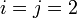$~i = j = 2$,$~0$$~=$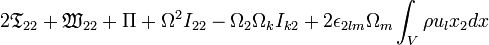$~ 2 \mathfrak{T}_{22} + \mathfrak{W}_{22} + \Pi + \Omega^2 I_{22} - \Omega_2\Omega_k I_{k2} + 2\epsilon_{2lm}\Omega_m \int_V \rho u_lx_2 dx$$~=$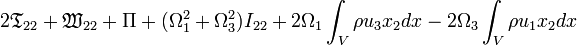$~ 2 \mathfrak{T}_{22} + \mathfrak{W}_{22} + \Pi + (\Omega_1^2 + \Omega_3^2) I_{22} + 2\Omega_1 \int_V \rho u_3x_2 dx - 2\Omega_3 \int_V \rho u_1x_2 dx$

and, for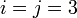$~i=j=3$,$~0$$~=$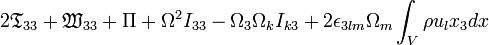$~ 2 \mathfrak{T}_{33} + \mathfrak{W}_{33} + \Pi + \Omega^2 I_{33} - \Omega_3\Omega_k I_{k3} + 2\epsilon_{3lm}\Omega_m \int_V \rho u_lx_3 dx$$~=$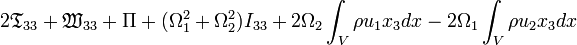$~ 2 \mathfrak{T}_{33} + \mathfrak{W}_{33} + \Pi + (\Omega_1^2 + \Omega_2^2) I_{33} + 2\Omega_2 \int_V \rho u_1x_3 dx - 2\Omega_1 \int_V \rho u_2 x_3 dx$

### The Six Off-Diagonal Elements

Notice that the off-diagonal components of both$~I_{ij}$ and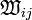$~\mathfrak{W}_{ij}$ are zero. Hence, the equilibrium expression that is dictated by each off-diagonal component of the 2nd-order TVE is,$~0$$~=$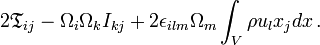$~ 2 \mathfrak{T}_{ij} - \Omega_i\Omega_k I_{kj} + 2\epsilon_{ilm}\Omega_m \int_V \rho u_lx_j dx \, .$

For example — as is explicitly illustrated on p. 130 of EFE — for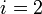$~i=2$ and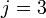$~j=3$,$~0$$~=$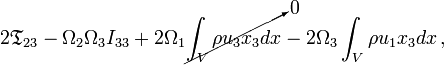$~ 2 \mathfrak{T}_{23} - \Omega_2\Omega_3 I_{33} + 2\Omega_1 \cancelto{0}{\int_V \rho u_3x_3 dx} - 2\Omega_3 \int_V \rho u_1x_3 dx \, ,$ [ EFE, Chapter 7, §47, Eq. (3) ]

whereas for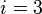$~i=3$ and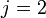$~j=2$,$~0$$~=$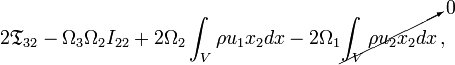$~ 2 \mathfrak{T}_{32} - \Omega_3 \Omega_2 I_{22} + 2\Omega_2 \int_V \rho u_1x_2 dx - 2\Omega_1 \cancelto{0}{\int_V \rho u_2 x_2 dx} \, ,$ [ EFE, Chapter 7, §47, Eq. (4) ]

where in both cases we have acknowledged that, given the above specific prescription for the internal velocity field,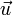$~\vec{u}$,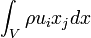$~\int_V \rho u_i x_j dx$$~=$$~0$ if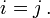$~i = j \, .$ [ EFE, Chapter 7, §47, Eq. (5) ]

Describe …

Describe …

Describe …